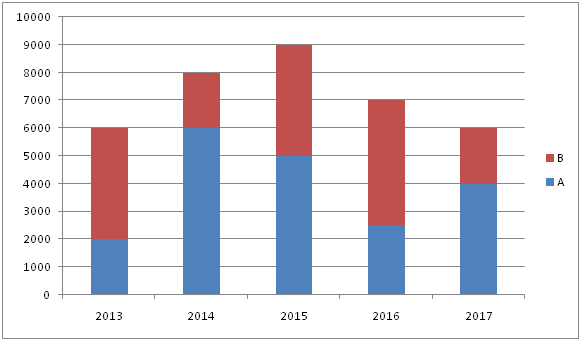# SBI Clerk Pre Quantitative Aptitude (Day-43)

Dear Aspirants, Our IBPS Guide team is providing new series of Quantitative Aptitude Questions for SBI Clerk Prelims 2020 so the aspirants can practice it on a daily basis. These questions are framed by our skilled experts after understanding your needs thoroughly. Aspirants can practice these new series questions daily to familiarize with the exact exam pattern and make your preparation effective.

Start Quiz

Ensure Your Ability Before the Exam – Take SBI Clerk 2020 Prelims Free Mock Test

Missing Number Series

Direction (1 – 5): What will come in place of question mark (?) in the following number series?

1) 56, 92, 67, 83, 74, ?

a) 90

b) 82

c) 78

d) 84

e) 93

2) 5040, 840, 168, ?, 14, 7

a) 38

b) 40

c) 42

d) 44

e) 46

3) 240, 40, ?, 50, 150, 75

a) 180

b) 200

c) 260

d) 280

e) 320

4) 12, 6, 12, 42, 210, ?

a) 1362

b) 1364

c) 1365

d) 1368

e) None of these

5) 71, 77, 89, 109, 139, ?

a) 180

b) 178

c) 179

d) 181

e) 177

Data Interpretation

Direction (6 – 10): Study the following information carefully and answer the given questions?

The given bar graph shows the investments of two partners A and B over the year.6) A and B started the business with them investment in 2014 and after 4 months C joined with investment of Rs. 2000. At the end of one year the total profit is Rs. 4200, then find B’s profit share?

a) Rs.900

b) Rs.600

c) Rs.1200

d) Rs.800

e) None of these

7) What is the average investment of B in all the years together?

a) Rs.2900

b) Rs.3000

c) Rs.3300

d) Rs.3900

e) None of these

8) C and D started the business with investment ratio of 4:5 and C’s investment is equal to the A’s investment in 2017. After one year B joined with his investment amount in 2017. At the end of three years, B’s profit share is Rs. 2000, what is the total profit?

a) Rs.12500

b) Rs.13500

c) Rs.14500

d) Rs.15500

e) None of these

9) Investment of A in 2016 is what percent of the investment of B in 2015?

a) 62.5%

b) 57.5%

c) 48.5%

d) 37.5%

e) None of these

10) What is the difference between the average investment of A in all the years together and the investment of B in 2016 and 2013 together?

a) Rs.4200

b) Rs.4600

c) Rs.5000

d) Rd.5400

e) None of these

Directions (1-5):

56 + 62=92

92 – 52 =67

67 + 42 =83

83 – 32 =74

74 + 22 =78

5040 ÷ 6=840

840 ÷ 5=168

168 ÷ 4=42

42 ÷ 3=14

14 ÷ 2=7

240 ÷ 6 =40

40 * 5 =200

200 ÷ 4 =50

50 * 3 =150

150 ÷ 2 =75

12 * 0.5=6

6 * 2 =12

12 * 3.5=42

42 * 5=210

210 * 6.5=1365

71 + (22 + 2) =77

77 + (32 + 3) =89

89 + (42 + 4) =109

109 + (52 + 5)=139

139 + (62 + 6)=181

Directions (6-10) :

Profit ratio

=6000 * 12 : 2000 * 12 : 2000 * 8

= 9:3:2

B’s share = 3/14 * 4200 = 900

Average of B’s investment

= (4000 + 2000 + 4000 + 4500 + 2000)/5 = 3300

C’s investment=4000

D’s investment=5000

B’s investment=2000

Profit ratio

=4000 * 3 :5000 * 3 : 2000 *2

=12:15:4

Total profit=31/4 * 2000 =15500

Required % = 2500/4000 * 100 = 62.5%

Average of A

= (2000 + 6000 + 5000 + 2500 + 4000)/5 = 3900

B in 2016 and 2013

= 4000 + 4500 = 8500

Difference = 8500 – 3900 = 4600

 Check Here to View SBI Clerk Prelims 2020 Quantitative Aptitude Questions Day 42 Day 41 Day 40 Click Here for SBI Clerk 2020 – Detailed Exam Notification